# ISEE Upper Level Math : How to add exponents

## Example Questions

← Previous 1

### Example Question #1 : How To Add Exponents

Simplify the expression: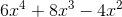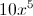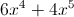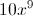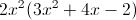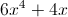Explanation: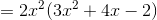### Example Question #2 : How To Add Exponents

Simplify: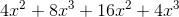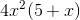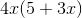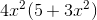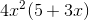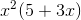Explanation:

In order to add exponential terms, both the base and the exponent must be the same. So we can write: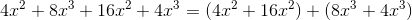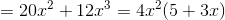### Example Question #3 : How To Add Exponents

Evaluate: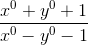Explanation:

Based on the zero-exponent rule we have: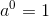That means any non-zero number raised to the zero power is equal to. So we can write: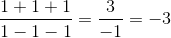### Example Question #4 : How To Add Exponents

What is the value of the expression: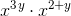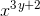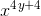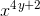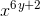Explanation:

When values, having the same base, are multiplied by one another, the exponents are added together and the base stays the same.

Thus,is equal to### Example Question #5 : How To Add Exponents

Simplify: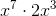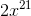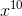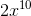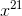Explanation:

When multiplying exponents, the exponents are added together. Thus, 3 and 7 are added together for a sum of 10. In this problem, the "2" becomes a coefficient in front of the x. Therefore, the correct answer is:### Example Question #6 : How To Add Exponents

Simplify: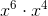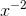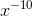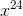Explanation:

When multiplying exponents, the exponents are added together.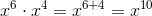### Example Question #7 : How To Add Exponents

Simplify: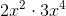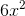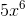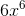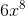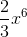Explanation:

When multiplying exponents, the exponents are added together.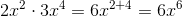### Example Question #8 : How To Add Exponents

Simplify the expression: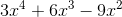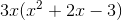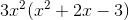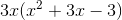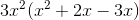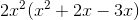Explanation:

To simplify this problem we need to factor out a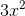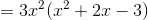We can do this because multiplying exponents is the same as adding them. Therefore,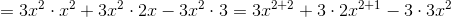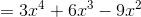### Example Question #9 : How To Add Exponents

Solve: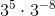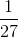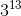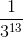Explanation:

It is not necessary to evaluate both terms and multiply.

According to the rules of exponents, when we have the same bases raised to some power that are multiplied with each other, we can add the powers.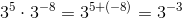This term can be rewritten as a fraction.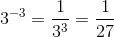The answer is:### Example Question #10 : How To Add Exponents

Which of the following is equivalent to the expression below?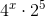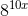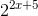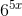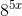Explanation:

When exponents are multiplied by one another, and the base is the same, the exponents can be added together.

The first step is to try to create a common base.Given that the square of 2 is for, the expression can be rewritten as: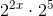← Previous 1